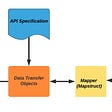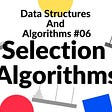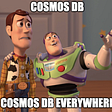# Solve state space equation by ODE45

Hello everybody.

I have state space equation for IM motor like this:

xdot=A.x+B.u → and their dimensions are : [50,1]=[50,50]*[50,1]+[50,50]*[50,1]

I have calculated A & B and I need to get xdot. (there are no C & D)

1- how should I write my function? (I don’t use Simulink)

2- I used my solver like this:

>> [t,y] = ode45(@sys, tspan, zeros(50,1));

NOTE:-

Matlabsolutions.com provide latest MatLab Homework Help,MatLab Assignment Help for students, engineers and researchers in Multiple Branches like ECE, EEE, CSE, Mechanical, Civil with 100% output.Matlab Code for B.E, B.Tech,M.E,M.Tech, Ph.D. Scholars with 100% privacy guaranteed. Get MATLAB projects with source code for your learning and research.

In order to solve an ODE using ode45, you need to first define the function to describe the complete dynamics. In the linear state space system you provided, the definition of u is missing. In general, u can be designed as a linear feedback control law, such as u = Kx, where K is a 50-by-50 matrix. Let me give you a simpler example here. Suppose we have A = [0 1; -2 3]; B = [0;1]; K = [-1 -1]; Then, the system function can be constructed as:

`function  dx = sys(t, x)   A = [0 1; -2 3]; B = [0;1]; K = [-1 -1];    u = K*x   dx = A*x + B*u;end`

Please save the above function in an MATLAB file and name is as sys.m

Then, in a separate MATLAB script file or in the command window, you can simulate the system by executing the following

--

--

--

## More from Technical Source

Simple! That is me, a simple person. I am passionate about knowledge and reading. That’s why I have decided to write and share a bit of my life and thoughts to.

Love podcasts or audiobooks? Learn on the go with our new app.

## IoT Based Salesforce Cloud## Using Python and Selenium To Scrape Facebook Fan Page Users## N.O.W w/ Mark11 and DKD## The Simplest Debugging Strategy That Many Beginners Ignore## Avoid exposing JPA entities in REST API, Instead generate client beans using OpenAPI Generator and…## Selection Algorithms## On Notation: writing music with Sonic Pi## Partitions in Cosmos DB## Technical Source

Simple! That is me, a simple person. I am passionate about knowledge and reading. That’s why I have decided to write and share a bit of my life and thoughts to.

## Pleasure and pain of running Neural networks on iPhone## What does Cost Function do in Machine learning.